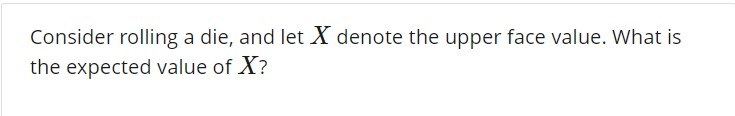# Consider rolling a die, and let X denote the upper face value. What isthe expected value of X?

Question
13 views

Hello. Can I get help on how to work out this problem? If there is also a way to calculate using the TI-83 graphing calculator, that would be helpful. Thanks!help_outlineImage TranscriptioncloseConsider rolling a die, and let X denote the upper face value. What is the expected value of X? fullscreen
check_circle

star
star
star
star
star
1 Rating
Step 1

Conditions to construct a probability distribution table:

Discrete random variable:

If the variable X takes finite or countable values, then the variable X is said to be discrete.

Conditions for the discrete probability distribution:

• The probabilities of all the values of the variable X must lie between 0 and 1.
• The sum of probabilities of all the values of X must be equal to “1”.
Step 2

Construct the probability distribution table for the upper face value of rolled die.

Consider that the variable X represents the upper face value of the rolled die.

Here, it is given that the six sided die is rolled. The numbers on the six sided die are 1, 2, 3, 4, 5 and 6.

That is, the variable X takes the values 1, 2, 3, 4, 5 and 6.

Since, the die is unbiased and total number of outcomes is 6, the probability of getting any value (1, 2, 3,4 5, 6) is p = 1/6.

Therefore, each value of the upper face of the rolled die will have equal chance of occurrence 1/6.

The probability distribution table for the upper face value of rolled die is obtained below:

Step 3

Find the expected value upper face value of the rolled die X:

The general formula to obtain the expected value of a discrete random variable is,

E(X) = ∑X*P(X)

The expected va...

### Want to see the full answer?

See Solution

#### Want to see this answer and more?

Solutions are written by subject experts who are available 24/7. Questions are typically answered within 1 hour.*

See Solution
*Response times may vary by subject and question.
Tagged in

### Statistics